# SET THEORY Set Notation Set is a collection

• Slides: 25
Download presentation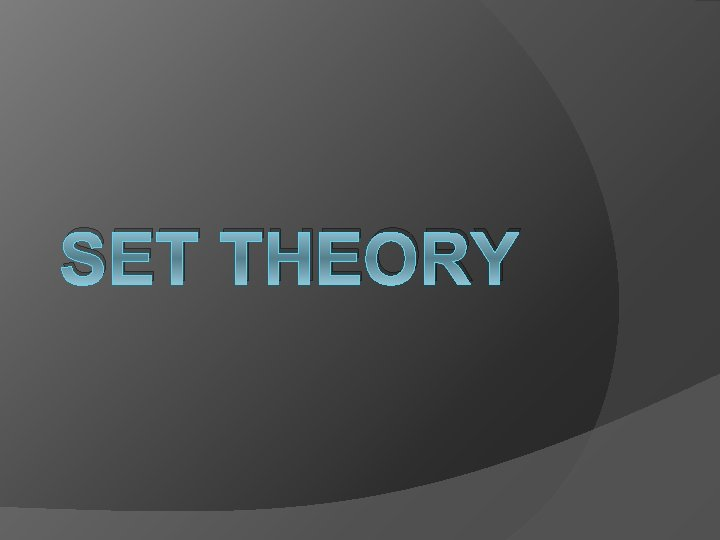SET THEORY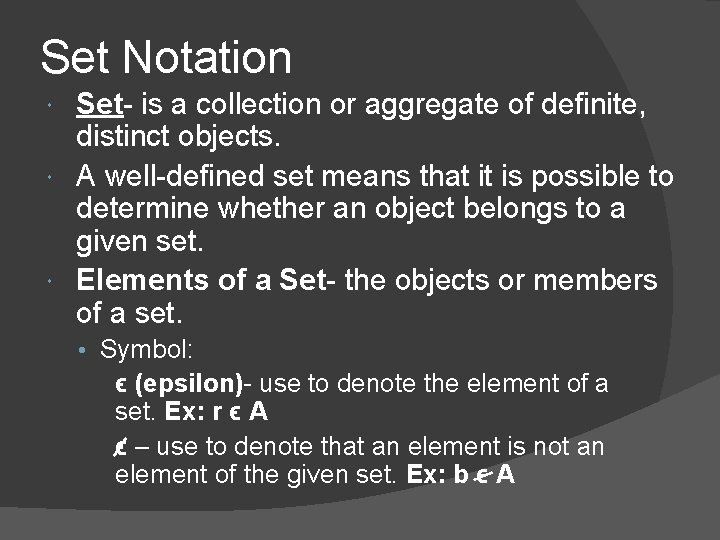Set Notation Set- is a collection or aggregate of definite, distinct objects. A well-defined set means that it is possible to determine whether an object belongs to a given set. Elements of a Set- the objects or members of a set. • Symbol: ϵ (epsilon)- use to denote the element of a set. Ex: r ϵ A ϵ – use to denote that an element is not an element of the given set. Ex: b ϵ A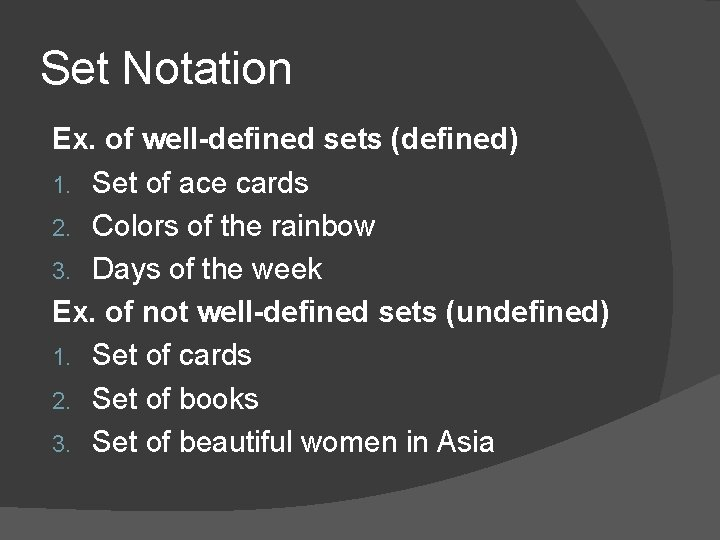Set Notation Ex. of well-defined sets (defined) 1. Set of ace cards 2. Colors of the rainbow 3. Days of the week Ex. of not well-defined sets (undefined) 1. Set of cards 2. Set of books 3. Set of beautiful women in AsiaSet Notation Different symbols are used when dealing with sets: 1. A pair of braces { } – is used to represent the idea of a set. 2. Capital letters of the English alphabet – are used to name sets. Example: A = { a, b, c, d, e } B = { 2, 4, 6, 8, 10 } Letters with subscripts can also be used, e. g. A 1, A 2, A 3, …etc.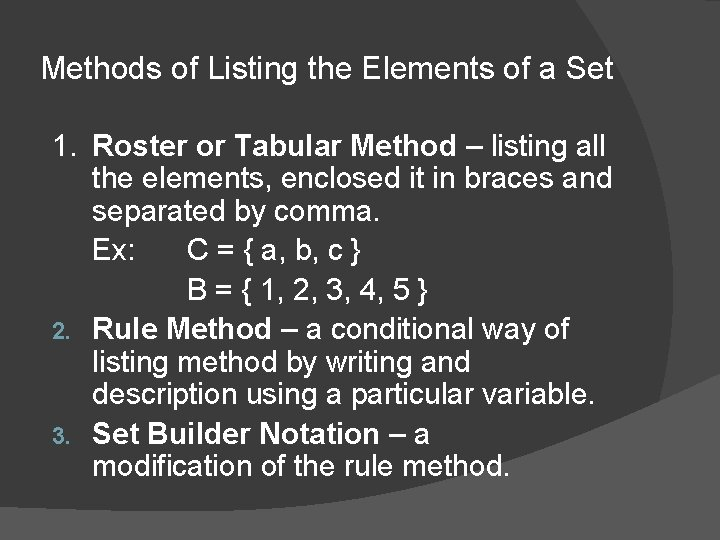Methods of Listing the Elements of a Set 1. Roster or Tabular Method – listing all the elements, enclosed it in braces and separated by comma. Ex: C = { a, b, c } B = { 1, 2, 3, 4, 5 } 2. Rule Method – a conditional way of listing method by writing and description using a particular variable. 3. Set Builder Notation – a modification of the rule method.Methods of Listing the Elements of a Set Examples: Rule Set-builder 1. A = { counting numbers less than 5} A = { x/x is a counting number less than 5} 2. B = { days of the week that begin with letter S } B = { d/d is a day of the week beginning with letter S } v The symbol ( / ) means “wherein” or “such that. ”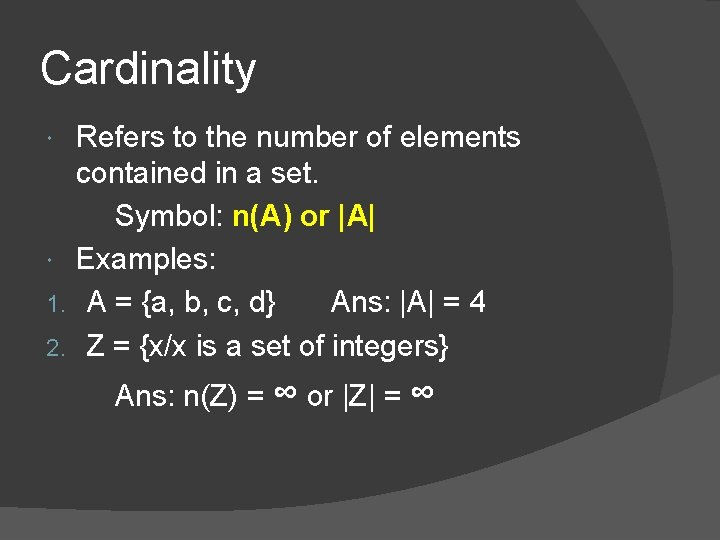Cardinality Refers to the number of elements contained in a set. Symbol: n(A) or |A| Examples: 1. A = {a, b, c, d} Ans: |A| = 4 2. Z = {x/x is a set of integers} Ans: n(Z) = ∞ or |Z| = ∞Kinds of SetsSubset – it is a part of a given set. Let A and B be sets. B is a subset of A if each element of B is an element of A. In symbols: B ⊂ A or B ⊆ A read as “B is a subset of A. ” Two kinds of subset: 1. Proper Subset – a part of a set, symbol ( ⊂ ) 2. Improper (Strict) Subset – the given set is equal to that set, symbol ( ⊆ )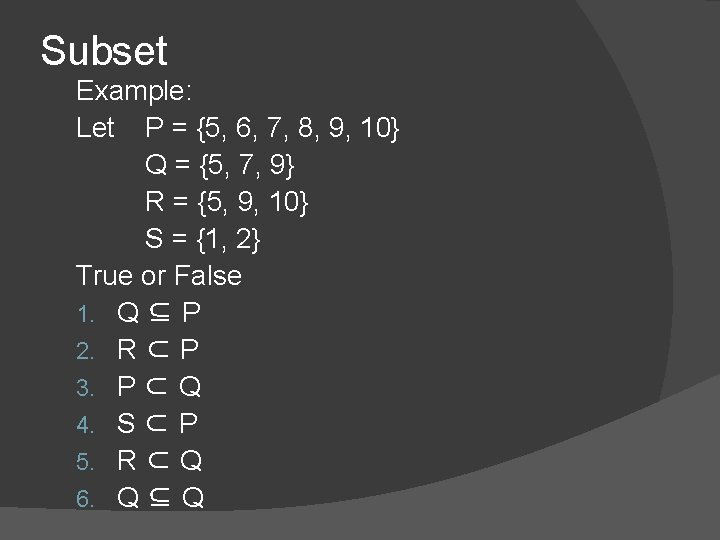Subset Example: Let P = {5, 6, 7, 8, 9, 10} Q = {5, 7, 9} R = {5, 9, 10} S = {1, 2} True or False 1. Q ⊆ P 2. R ⊂ P 3. P ⊂ Q 4. S ⊂ P 5. R ⊂ Q 6. Q ⊆ Q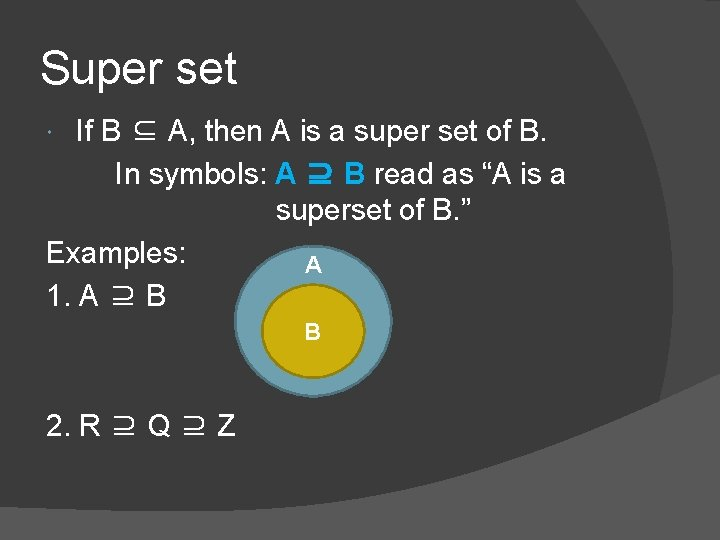Super set If B ⊆ A, then A is a super set of B. In symbols: A ⊇ B read as “A is a superset of B. ” Examples: A 1. A ⊇ B B 2. R ⊇ Q ⊇ ZKinds of Sets Empty or Null Set – sets having no elements. Symbol: { } or ∅ Note: An empty set is a subset of any set. Ø Universal Set (U) – also called the general set, is the sum of all sets or the totality of elements under consideration or a particular discussion. Example: U = {a, b, c, d, x, y, z} A = {a, b, c, d} B = {x, y, z} ØKinds of Sets Unit Set – set having only one element. Ex: D = {y/y is a day of the week that begins with letter M} Ø Finite Set – sets having a limited or countable number of elements. Ex: set of counting numbers less than 5 set of colors of the rainbow Ø Infinite Set – sets having an unlimited or uncountable number of elements. Ex: set of counting numbers Ø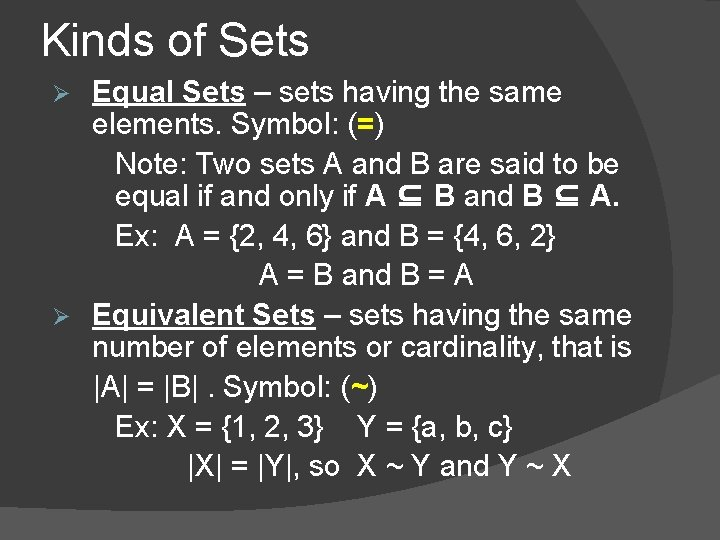Kinds of Sets Equal Sets – sets having the same elements. Symbol: (=) Note: Two sets A and B are said to be equal if and only if A ⊆ B and B ⊆ A. Ex: A = {2, 4, 6} and B = {4, 6, 2} A = B and B = A Ø Equivalent Sets – sets having the same number of elements or cardinality, that is |A| = |B|. Symbol: (~) Ex: X = {1, 2, 3} Y = {a, b, c} |X| = |Y|, so X ~ Y and Y ~ X Ø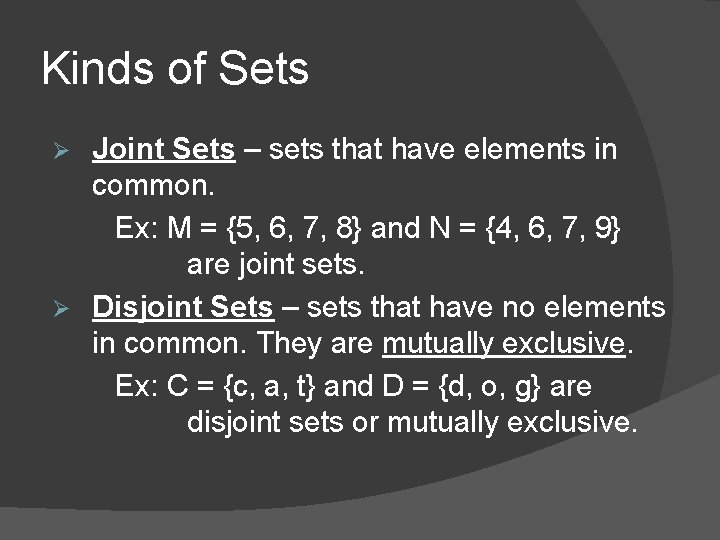Kinds of Sets Joint Sets – sets that have elements in common. Ex: M = {5, 6, 7, 8} and N = {4, 6, 7, 9} are joint sets. Ø Disjoint Sets – sets that have no elements in common. They are mutually exclusive. Ex: C = {c, a, t} and D = {d, o, g} are disjoint sets or mutually exclusive. Ø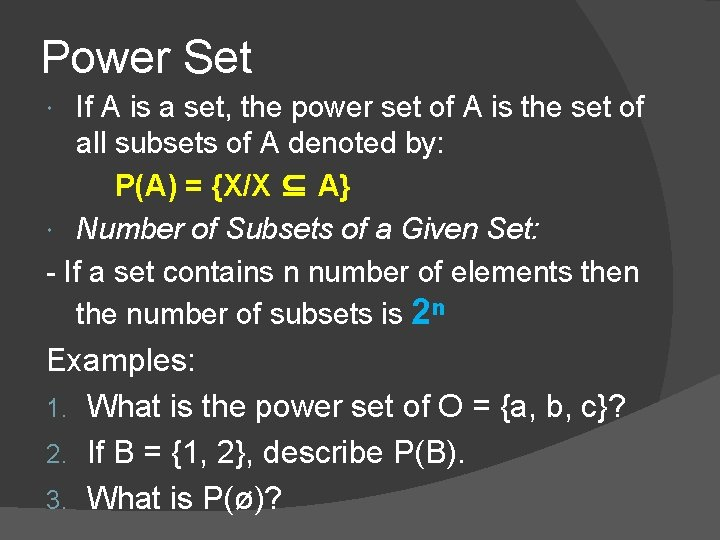Power Set If A is a set, the power set of A is the set of all subsets of A denoted by: P(A) = {X/X ⊆ A} Number of Subsets of a Given Set: - If a set contains n number of elements then the number of subsets is 2ⁿ Examples: 1. What is the power set of O = {a, b, c}? 2. If B = {1, 2}, describe P(B). 3. What is P(ø)?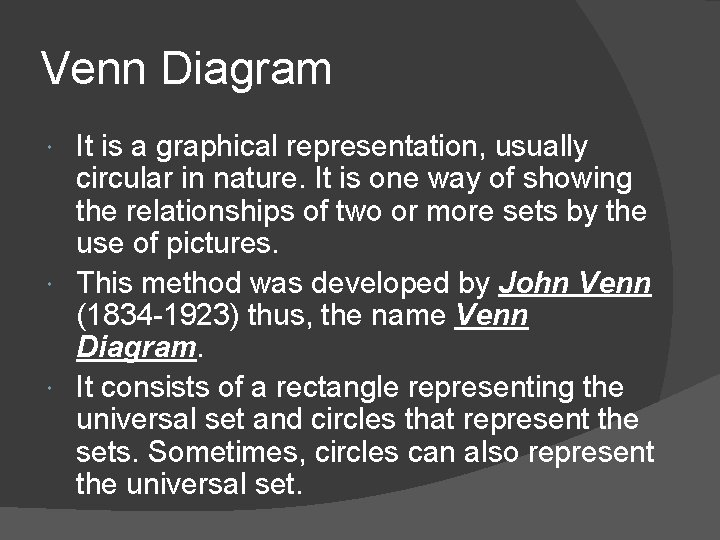Venn Diagram It is a graphical representation, usually circular in nature. It is one way of showing the relationships of two or more sets by the use of pictures. This method was developed by John Venn (1834 -1923) thus, the name Venn Diagram. It consists of a rectangle representing the universal set and circles that represent the sets. Sometimes, circles can also represent the universal set.Venn Diagram U A B a, b, c d, e, f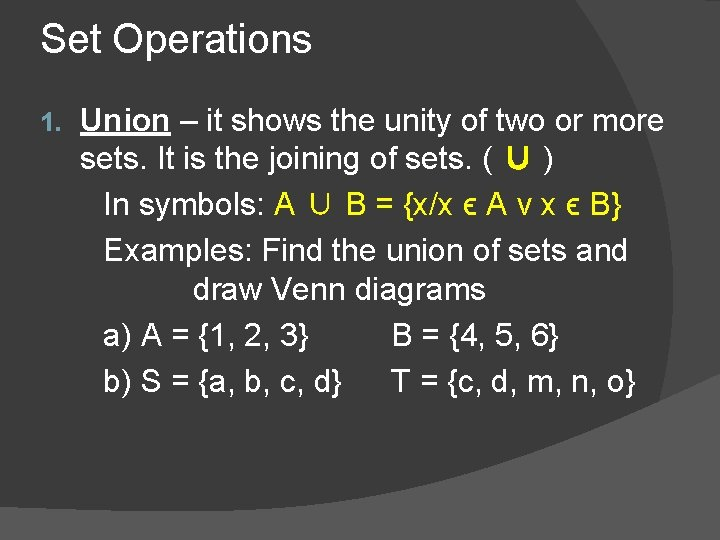Set Operations 1. Union – it shows the unity of two or more sets. It is the joining of sets. ( ∪ ) In symbols: A ∪ B = {x/x ϵ A v x ϵ B} Examples: Find the union of sets and draw Venn diagrams a) A = {1, 2, 3} B = {4, 5, 6} b) S = {a, b, c, d} T = {c, d, m, n, o}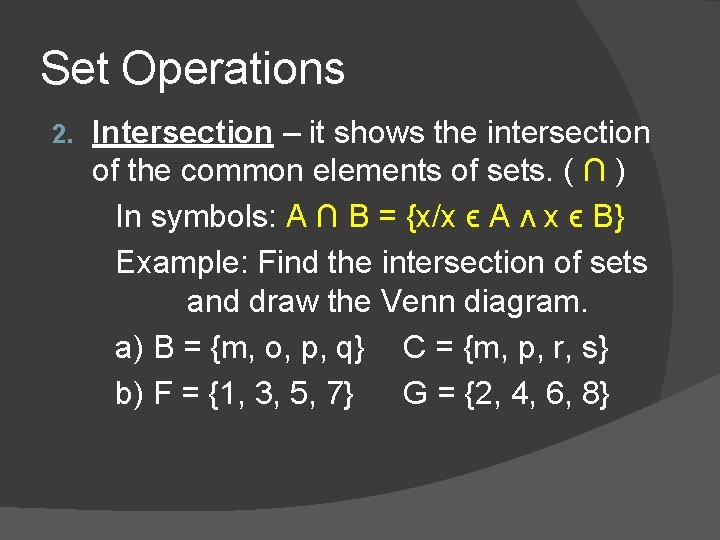Set Operations 2. Intersection – it shows the intersection of the common elements of sets. ( ∩ ) In symbols: A ∩ B = {x/x ϵ A ʌ x ϵ B} Example: Find the intersection of sets and draw the Venn diagram. a) B = {m, o, p, q} C = {m, p, r, s} b) F = {1, 3, 5, 7} G = {2, 4, 6, 8}Set Operations 3. Complement of a Set – it is the set whose elements are in the universal set but not in a set or a given set. ( ʼ ) In symbols: A’ = {x ϵ U / x ϵ A} Ex: Let U be the universal set. U = {2, 4, 6, 8, 10} A = {6, 8, 10} B = {2, 4, 6} Find: a) A’ b) B’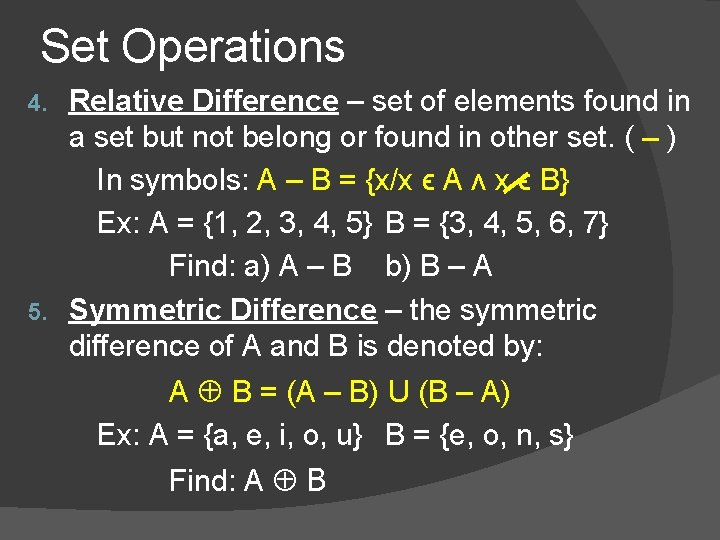Set Operations Relative Difference – set of elements found in a set but not belong or found in other set. ( – ) In symbols: A – B = {x/x ϵ A ʌ x ϵ B} Ex: A = {1, 2, 3, 4, 5} B = {3, 4, 5, 6, 7} Find: a) A – B b) B – A 5. Symmetric Difference – the symmetric difference of A and B is denoted by: 4. A B = (A – B) U (B – A) Ex: A = {a, e, i, o, u} B = {e, o, n, s} Find: A B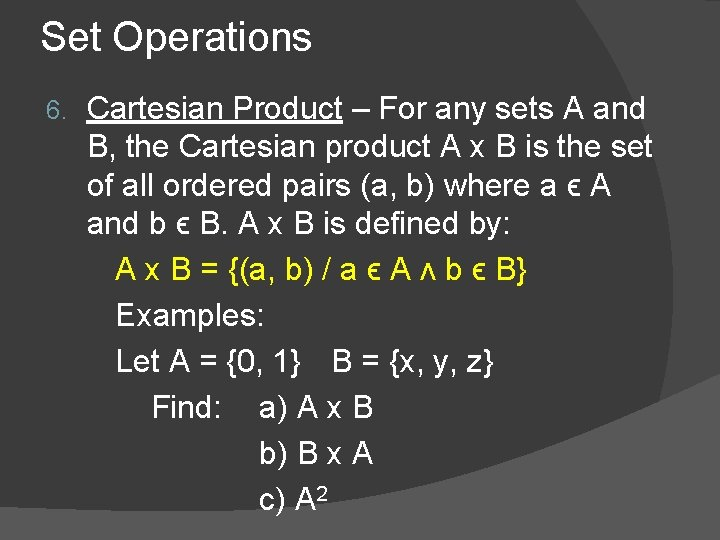Set Operations 6. Cartesian Product – For any sets A and B, the Cartesian product A x B is the set of all ordered pairs (a, b) where a ϵ A and b ϵ B. A x B is defined by: A x B = {(a, b) / a ϵ A ʌ b ϵ B} Examples: Let A = {0, 1} B = {x, y, z} Find: a) A x B b) B x A c) A 2Exercises Given: Let U be the universal set U = {a, b, c, d, e, f, g, h} A = (a, b, c} C = {a, g, h} B = {d, e, f} D = { b, c, d, e, f} Find the ff. and draw Venn diagrams. 1) A’ ∩ B’ 6) P(A) 2) (A U B)’ 7) B x C 3) (C’ ∩ D) U A 8) A – C 4) (A ∩ B)’ 9) D – B 5) A’ U B’ 10) A DApplications of Set Theory Examples 1. In a class, 15 are taking English, 20 are taking Filipino and 10 are taking both English and Filipino. How many students are there in all? 2. Of 1000 applicants for a mountain-climbing trip in the Himalayas, 450 get altitude sickness, 622 are not in good enough shape, and 30 have allergies. An applicant qualifies if and only if this applicant does not get altitude sickness, is in good shape, and does not have allergies. If there are 111 applicants who get altitude sickness and are not in good enough shape, 14 who get altitude sickness and have allergies, 18 who are not in good enough shape and have allergies, and 9 who get altitude sickness, are not in good enough shape, and have allergies, how many applicants qualify?